## Saturday, 9 August 2014

### CHAPTER 5 - Worked Out Examples 3

 Example: 5
 How will you expand the multinomial expression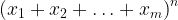${\left( {{x_1} + {x_2} +\ldots + {x_m}} \right)^n}$?
 Solution: 5
We will approach this problem using combinatorics. Note that a general term of the expansion would be of the form (without the coefficient)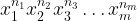$x_1^{{n_1}}x_2^{{n_2}}x_3^{n{ _3}}\ldots x_m^{{n_m}}$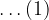$\ldots(1)$
where the various powers must always sum to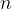$n$ (why?).
i.e.,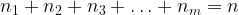${n_1} + {n_2} + {n_3} + \ldots + {n_m} = n$
Now, to evaluate the coefficient of the term in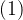$(1)$, we consider the multinomial expression in expanded form: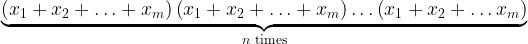$\underbrace {\left( {{x_1} + {x_2} + \ldots + {x_m}} \right)\left( {{x_1} + {x_2} +\ldots + {x_m}} \right) \ldots \left( {{x_1} + {x_2} +\ldots {x_m}} \right)}_{n\,\,{\rm{times}}}$
To generate the term in$(1)$, we must get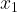${x_1}$ from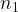${n_1}$ terms,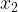${x_2}$ from${n_1}$ terms and so on. Let us find the number of ways in which this can be done.
First select those${n_1}$ multinomials that will contribute${x_1}$ : this can be done in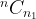${}^n{C_{{n_1}}}$ ways. Now, from the remaining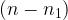$\left( {n - {n_1}} \right)$ multinomials, select those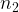${n_2}$ multinomials that will contribute${x_2}$ : this can be done in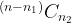${}^{(n - {n_1})}{C_{{n_2}}}$ ways. Continuing this process, we see that the number of ways to get${x_1}$ from${n_1}$${x_2}$ from${n_2} \ldots$ and so on, that is, the number of times the term in$(1)$ will be generated in the expansion is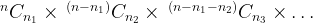$^n{C_{{n_1}}} \times {\,^{\left( {n - {n_1}} \right)}}{C_{{n_2}}} \times {\,^{\left( {n - {n_1} - {n_2}} \right)}}{C_{{n_3}}} \times\ldots$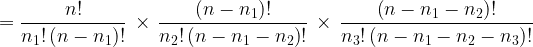$= \dfrac{{n!}}{{{n_1}!\left( {n - {n_1}} \right)!}}\,\, \times \,\,\dfrac{{\left( {n - {n_1}} \right)!}}{{{n_2}!\left( {n - {n_1} - {n_2}} \right)!}}\,\, \times \,\,\dfrac{{\left( {n - {n_1} - {n_2}} \right)!}}{{{n_3}!\left( {n - {n_1} - {n_2} - {n_3}} \right)!}}$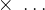$\,\, \times \,\,\ldots$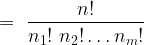$= \,\,\dfrac{{n!}}{{{n_1}!\,\,{n_2}!\ldots {n_m}!}}\,$
This is what is known as the general multinomial coefficient. The multinomial expansion can now be written compactly as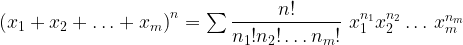${\left( {{x_1} + {x_2} + \ldots + {x_m}} \right)^n} = \sum {\dfrac{{n!}}{{{n_1}!{n_2}! \ldots {n_m}!}}} \,\,x_1^{{n_1}}x_2^{{n_2}}\ldots \,x_m^{{n_m}}$
where the summation is carried out over all possible combinations of the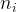${n_i}$‘s such that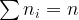$\sum {{n_i} = n}$. For example, in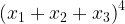${\left( {{x_1} + {x_2} + {x_3}} \right)^4}$, let us consider some terms in the expansion:
 Multinomial Term Coefficient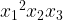${x_1}^2{x_2}{x_3}$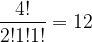$\dfrac{{4!}}{{2!1!1!}} = 12$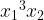${x_1}^3{x_2}$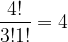$\dfrac{{4!}}{{3!1!}} = 4$${\left( {{x_1} + {x_2} + {x_3}} \right)^4}$${x_2}^4$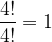$\dfrac{{4!}}{{4!}} = 1$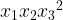${x_1}{x_2}{x_3}^2$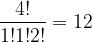$\dfrac{{4!}}{{1!1!2!}} = 12$$\vdots$
 Example: 6
 Find the coefficient of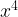${x^4}$ in the expansion of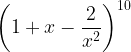${\left( {1 + x - \dfrac{2}{{{x^2}}}} \right)^{10}}$.
 Solution: 6
From the previous example, the general term in the expansion will be$\dfrac{{10!}}{{{n_1}!\;{n_2}!\;{n_3}!}}\;{(1)^{{n_1}}}{(x)^{{n_{^2}}}}{\left( {\dfrac{{ - 2}}{{{x^2}}}} \right)^{{n_{^3}}}}$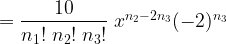$= \dfrac{{10}}{{{n_1}!\;{n_2}!\;{n_3}!}}\;{x^{{n_2} - 2{n_3}}}{( - 2)^{{n_3}}}$
where${n_1} + {n_2} + {n_3}$ must be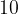$10$.
Now,${x^4}$ is generated whenever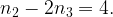${n_2} - 2{n_3} = 4.$ The possible values of the triplet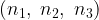$({n_1},\;{n_2},\;{n_3})$ can now simply be listed out: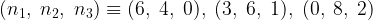$({n_1},\;{n_2},\;{n_3}) \equiv (6,\;4,\;0),\;(3,\;6,\;1),\;(0,\;8,\;2)$
Thus, the (total) coefficient of${x^4}$ is$\dfrac{{10!}}{{6!\;4!\;0!}}{( - 2)^0} + \dfrac{{10!}}{{3!\;6!\;1!}}{( - 2)^1} + \dfrac{{10!}}{{0!\;8!\;2!}}{( - 2)^2}$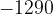$- 1290$ (verify)